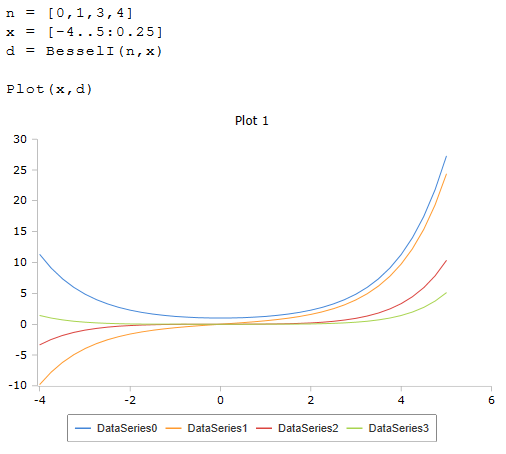# Besseli and Besselie Function

Modified Bessel function Iv(x) of the first kind

RedCrab Version 8.0

## Function Besseli

The function Besseli calculates the modified Bessel function Iv (x) of the first kind. The modified Bessel functions do not return an oscillating but an exponential behavior. A real or a complex number can be transferred as parameter x. A single value or a list can be passed.

Besseli (n, x)

## Function Besselie

The Besselie function differs from Besseli in that it uses the logarithmically scaled modified Bessel function for calculation A real or complex number, a single value or a list are also permitted as parameter x.

Besselie (n, x)

## Description

Single values or lists can be used as parameters n and x. The function is then calculated for each individual element of the list. The result is then a one-dimensional list, or a table if both parameters are lists.

If the real value of x is negative, the result can be a complex number.

### ExamplePlot of the Bessel function with the order numbers 0, 1, 3 and 4